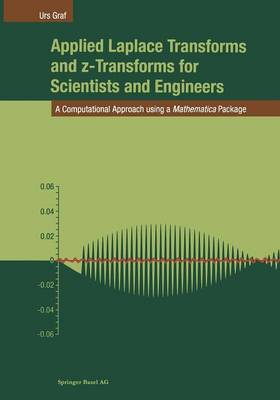•# Applied Laplace Transforms and z-Transforms for Scientists and Engineers: A Computational Approach using a Mathematica Package

(author)
£91.80
Mixed media product 500 Pages / Published: 24/05/2004
• We can order this

Usually dispatched within 3 weeks

The theory of Laplace transformation is an important part of the mathematical background required for engineers, physicists and mathematicians. Laplace transformation methods provide easy and effective techniques for solving many problems arising in various fields of science and engineering, especially for solving differential equations. What the Laplace transformation does in the field of differential equations, the z-transformation achieves for difference equations. The two theories are parallel and have many analogies. Laplace and z transformations are also referred to as operational calculus, but this notion is also used in a more restricted sense to denote the operational calculus of Mikusinski. This book does not use the operational calculus of Mikusinski, whose approach is based on abstract algebra and is not readily accessible to engineers and scientists. The symbolic computation capability of Mathematica can now be used in favor of the Laplace and z-transformations. The first version of the Mathematica Package LaplaceAndzTransforrns developed by the author appeared ten years ago. The Package computes not only Laplace and z-transforms but also includes many routines from various domains of applications. Upon loading the Package, about one hundred and fifty new commands are added to the built-in commands of Mathematica. The code is placed in front of the already built-in code of Laplace and z-transformations of Mathematica so that built-in functions not covered by the Package remain available. The Package substantially enhances the Laplace and z-transformation facilities of Mathematica. The book is mainly designed for readers working in the field of applications.

Publisher: Birkhauser Verlag AG
ISBN: 9783764324278
Number of pages: 500
Weight: 1200 g
Dimensions: 254 x 178 x 31 mm
Edition: 2004 ed.

MEDIA REVIEWS

"This book is a very good tool for scientists and engineers that work in the fields of applications, especially on practical problems leading to ordinary differential equations which could be solved through Laplace transformation."(ZENTRALBLATT MATH)

"This monograph gives an introduction to the Laplace and z-transformations with emphases on applications in engineering and mechanics. Throughout the book a Mathematics package developed by the authors is used, which substantially enhances the built facilities of Mathematics. Both analytical and numerical aspects are treated."

---MONATSHEFTE FUER MATHEMATIK